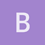Archive 04/01/2022.

Indel models: Why continuous time?liyu

Hi everyone! I have been doing research on the TKF91 model and its successors for over a year now. Recently, I have been tasked with writing a research proposal, on mathematical / statistical / computational aspects of indel models, for a general math-professor audience.

It seems that, for Markovian indel models at least, continuous-time models (TKF91, TKF92, long indel, Poisson indel, etc.) are much more popular than discrete-time models. (I only know of one discrete-time indel model, due to David Koslicki in his PhD thesis. And this work has only 7 citations.) I have been wondering why this is the case. Why have continuous-time models been so much more popular than discrete-time models? And relatedly, why did TKF choose continuous time over discrete time for their 1991 paper?

If I know that discrete-time models are actually valid, then I can avoid continuous-time models in my proposal (to make things less confusing to the reader). In addition, depending on the virtues of continuous time versus discrete time, it may make a good research proposal to study discrete-time indel models until we understand them as well as continuous-time indel models.

One possible argument for continuous time may be that continuous-time Markov chains are simpler than discrete-time countable-state Markov chains. For instance, in continuous time there are no periodicity issues (in continuous time, ergodic is equivalent to being both irreducible and positive-recurrent, whereas in discrete time for a countable state space, ergodic is equivalent to irreducible, aperiodic and positive-recurrent). However, most people (including me) would say discrete time is simpler than continuous time – the continuous-time theory has a few more technical complications.

Another possible argument may be that continuous-time models are more realistic. For instance, in continuous-time models we can have arbitrary branch lengths, whereas in discrete-time models branch lengths are multiples of a fixed constant. However, I see almost no problems with choosing the constant small enough so we can have a dense-enough range of possible branch lengths as we want.

So both arguments are unconvincing to me.ematsen

Interesting question!

Phylogenetic trees are typically parameterized with continuous branch lengths, which gives a point-mutation likelihood in terms of the standard CTMC. This makes it easy to also add a likelihood component from TKF91, etc.

How do you propose incorporating a discrete-time likelihood?liyu

To be honest, I did not understand much beginning with the phrase “which gives a point-mutation likelihood in terms of the standard CTMC”.

What “point-mutation likelihood” are you referring to? And what do you mean by “in terms of the standard CTMC”? I see that in the original TKF91 paper, equation (1) gives a likelihood expression in terms of the equilibrium distribution P_infinity and the transition probabilities P_t(A|C) of the CTMC.

And I don’t see why “this makes it easy to also add a likelihood component from TKF91”.

As for a discrete-time likelihood, maybe it will be just like in continuous time (equation (1) in TKF91 seems to work). (Not sure if that answers what you’re talking about.)

My proposal to “study discrete-time indel models until we understand them well” was motivated by the explicit formulas for the transition probabilities of TKF91. Maybe we can find explicit formulas in the discrete-time case as well (though I suspect someone else might have already done so and I just don’t know about their work).